# Selina Solutions Concise Mathematics Class 6 Chapter 29 The Circle Exercise 29(B)

Selina Solutions Concise Mathematics Class 6 Chapter 29 The Circle Exercise 29(B) has 100% accurate answers in a stepwise manner, for the convenience of students. Frequent practice of these solutions not only improves analytical and logical thinking abilities but also helps students to secure high marks in the annual exam. Solutions created by expert teachers, have important shortcut methods to solve tricky problems effortlessly. Students can clear their doubts immediately by themselves while revising exercise problems, using these solutions. For more conceptual knowledge, students can download Selina Solutions Concise Mathematics Class 6 Chapter 29 The Circle Exercise 29(B) PDF, from the links which are provided here.

## Selina Solutions Concise Mathematics Class 6 Chapter 29 The Circle Exercise 29(B) Download PDF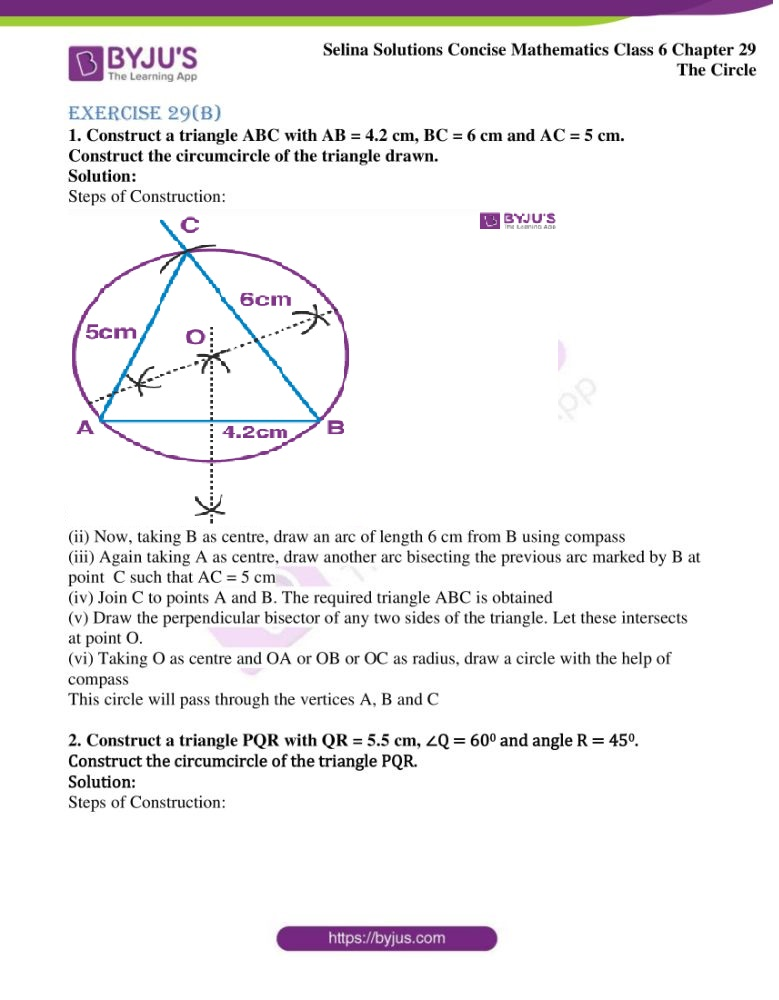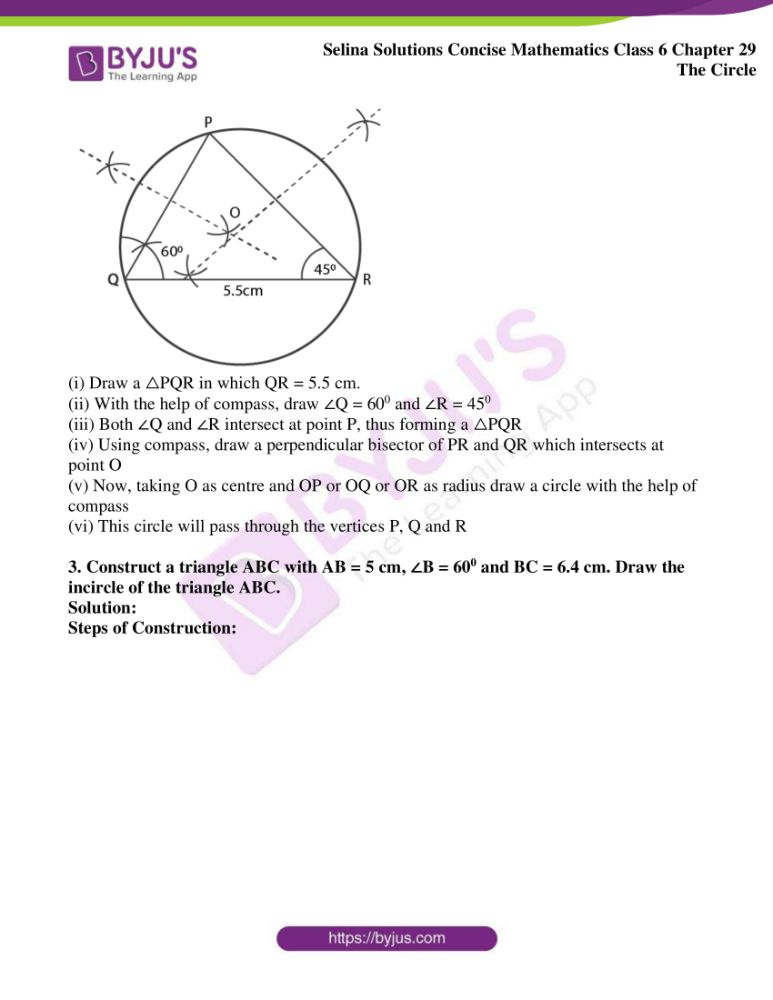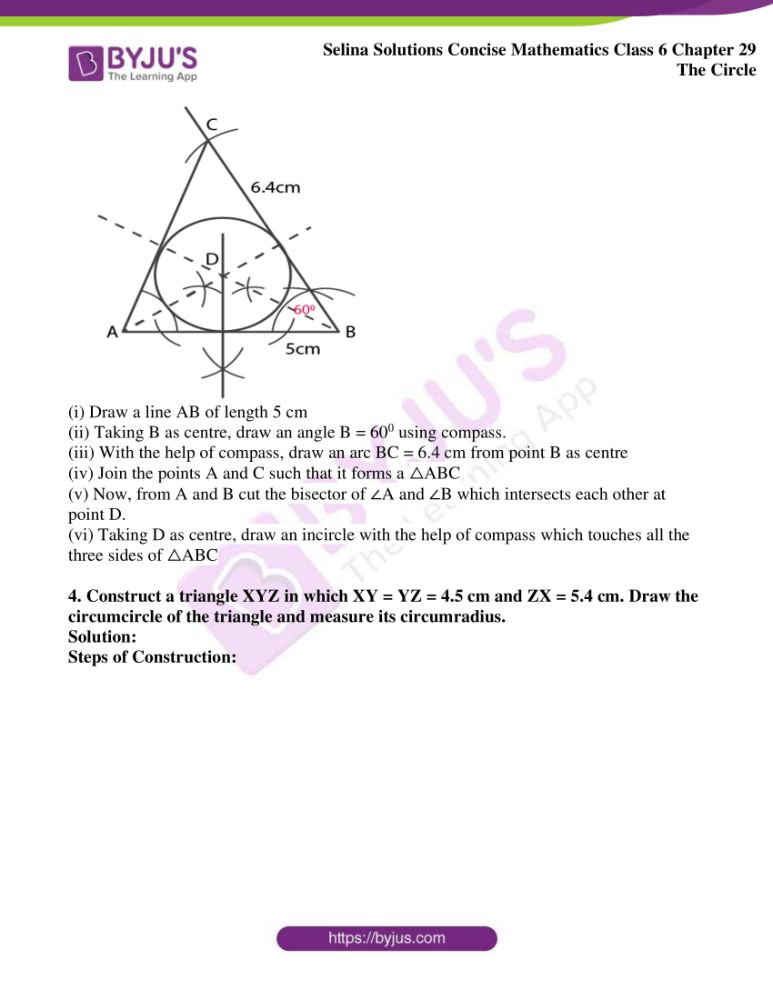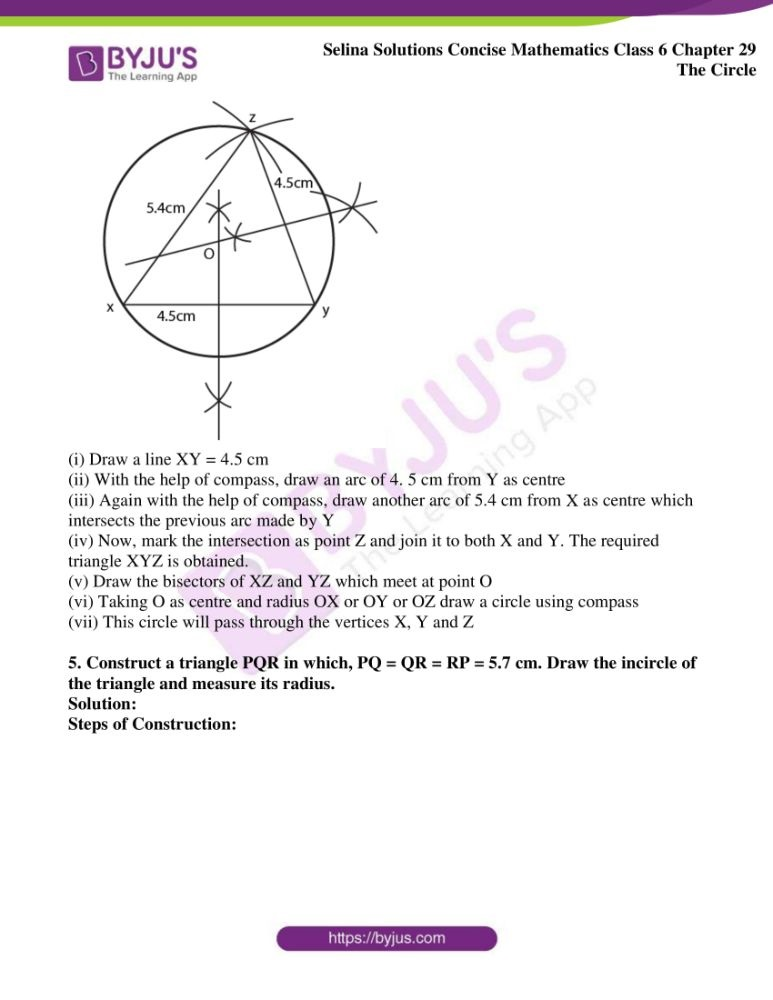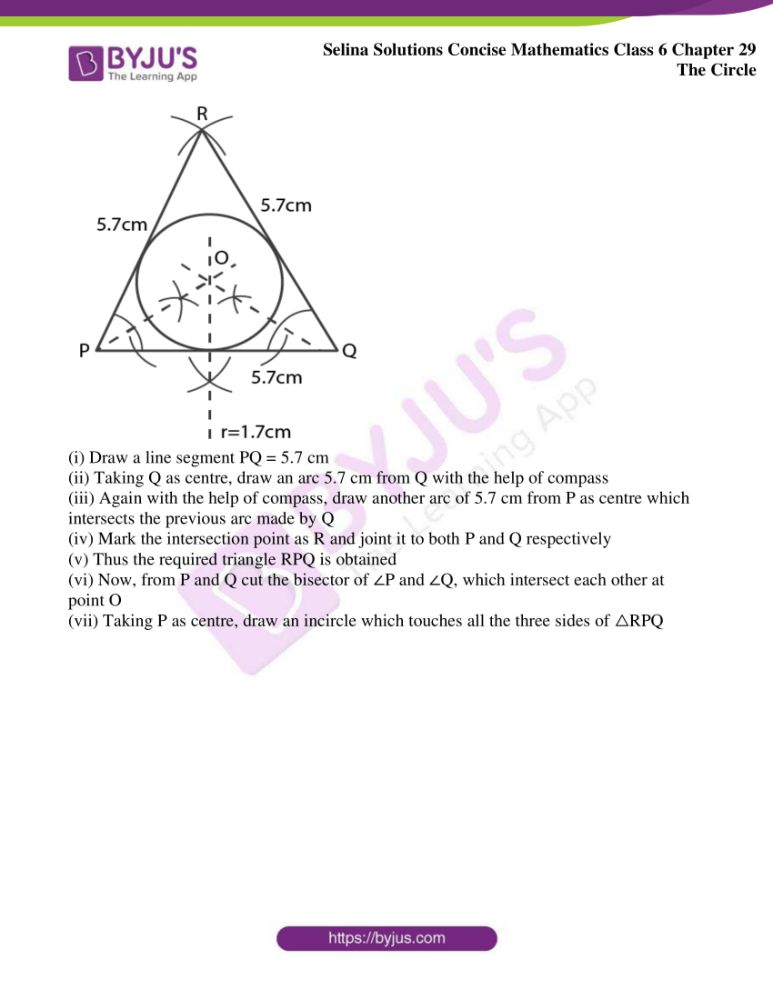### Access another exercise of Selina Solutions Concise Mathematics Class 6 Chapter 29 The Circle

Exercise 29(A) Solutions

## Access Selina Solutions Concise Mathematics Class 6 Chapter 29 The Circle Exercise 29(B)

Exercise 29(B)

1. Construct a triangle ABC with AB = 4.2 cm, BC = 6 cm and AC = 5 cm. Construct the circumcircle of the triangle drawn.

Solution:

Steps of Construction: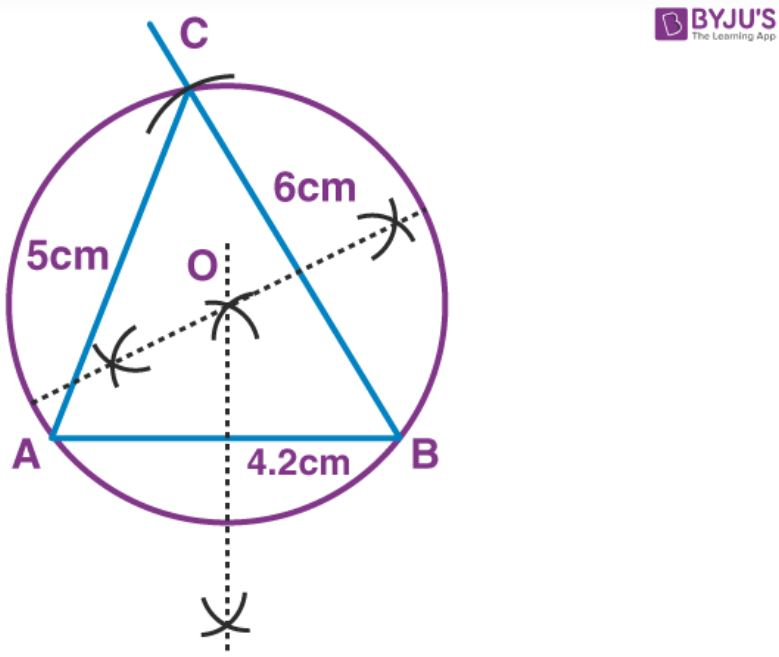(i) Draw a line segment AB = 4.2 cm

(ii) Now, taking B as centre, draw an arc of length 6 cm from B using compass

(iii) Again taking A as centre, draw another arc bisecting the previous arc marked by B at point C such that AC = 5 cm

(iv) Join C to points A and B. The required triangle ABC is obtained

(v) Draw the perpendicular bisector of any two sides of the triangle. Let these intersects at point O.

(vi) Taking O as centre and OA or OB or OC as radius, draw a circle with the help of compass

This circle will pass through the vertices A, B and C

2. Construct a triangle PQR with QR = 5.5 cm, ∠Q = 600 and angle R = 450. Construct the circumcircle of the triangle PQR.

Solution:

Steps of Construction: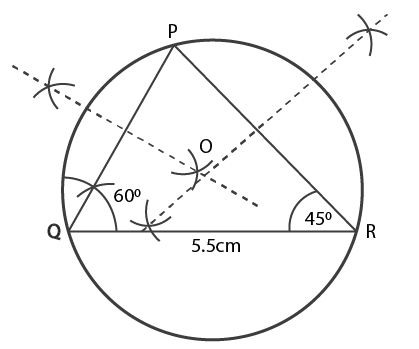(i) Draw a △PQR in which QR = 5.5 cm.

(ii) With the help of compass, draw ∠Q = 600 and ∠R = 450

(iii) Both ∠Q and ∠R intersect at point P, thus forming a △PQR

(iv) Using compass, draw a perpendicular bisector of PR and QR which intersects at point O

(v) Now, taking O as centre and OP or OQ or OR as radius draw a circle with the help of compass

(vi) This circle will pass through the vertices P, Q and R

3. Construct a triangle ABC with AB = 5 cm, ∠B = 600 and BC = 6.4 cm. Draw the incircle of the triangle ABC.

Solution:

Steps of Construction: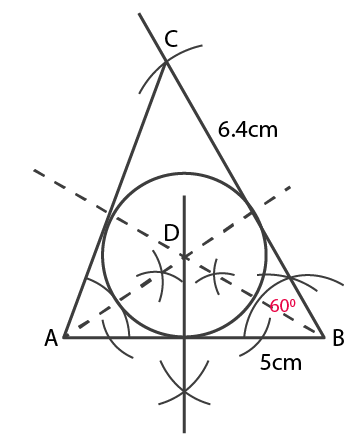(i) Draw a line AB of length 5 cm

(ii) Taking B as centre, draw an angle B = 600 using compass.

(iii) With the help of compass, draw an arc BC = 6.4 cm from point B as centre

(iv) Join the points A and C such that it forms a △ABC

(v) Now, from A and B cut the bisector of ∠A and ∠B which intersects each other at point D.

(vi) Taking D as centre, draw an incircle with the help of compass which touches all the three sides of △ABC

4. Construct a triangle XYZ in which XY = YZ = 4.5 cm and ZX = 5.4 cm. Draw the circumcircle of the triangle and measure its circumradius.

Solution:

Steps of Construction: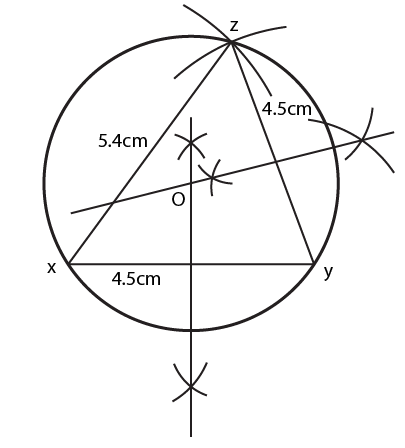(i) Draw a line XY = 4.5 cm

(ii) With the help of compass, draw an arc of 4. 5 cm from Y as centre

(iii) Again with the help of compass, draw another arc of 5.4 cm from X as centre which intersects the previous arc made by Y

(iv) Now, mark the intersection as point Z and join it to both X and Y. The required triangle XYZ is obtained.

(v) Draw the bisectors of XZ and YZ which meet at point O

(vi) Taking O as centre and radius OX or OY or OZ draw a circle using compass

(vii) This circle will pass through the vertices X, Y and Z

5. Construct a triangle PQR in which, PQ = QR = RP = 5.7 cm. Draw the incircle of the triangle and measure its radius.

Solution:

Steps of Construction: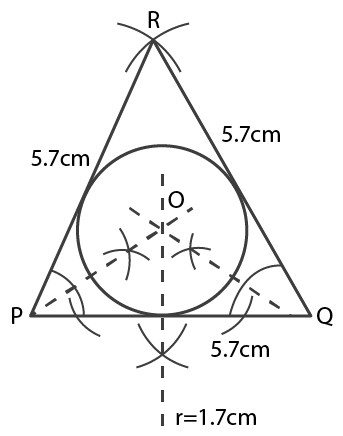(i) Draw a line segment PQ = 5.7 cm

(ii) Taking Q as centre, draw an arc 5.7 cm from Q with the help of compass

(iii) Again with the help of compass, draw another arc of 5.7 cm from P as centre which intersects the previous arc made by Q

(iv) Mark the intersection point as R and joint it to both P and Q respectively

(v) Thus the required triangle RPQ is obtained

(vi) Now, from P and Q cut the bisector of ∠P and ∠Q, which intersect each other at point O

(vii) Taking P as centre, draw an incircle which touches all the three sides of △RPQ